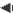voriger Punkt Kapitel 23: Zahlen nächster Punkt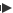23.2 Kardinalzahlen

 Kardinalzahlen1 = one 21 = twenty-one 2 = two 30 = thirty 3 = three 31 = thirty-one 4 = four 40 = forty 5 = five 50 = fifty 6 = six 60 = sixty 7 = seven 70 = seventy 8 = eight 80 = eighty 9 = nine 90 = ninety 10 = ten 100 = one hundred 11 = eleven 101 = one hundred and one 12 = twelve 200 = two hundred 13 = thirteen 256 = two hundred fifty-six 14 = fourteen 300 = three hundred 15 = fifteen 400 = four hundred 16 = sixteen 1000 = one thousand 17 = seventeen 10000 = ten thousand 18 = eighteen 100000 = one hundred thousand 19 = nineteen 1000000 = one million 20 = twenty 1000000000 = one thousand million (BE)                         one billion (AE)

Eigentlich ist das System ganz einfach. Für die -zehn wird -teen angehängt. Für die -zig wird -ty angehängt. Nur die Sache mit den Einern ist ein wenig ungewohnt für deutsche Ohren. Während wir sagen einundzwanzig, sagt man im Englischen zwanzig-eins (= twenty-one).voriger Punkt Kapitel 23: Zahlen nächster PunktKontakt Impressum Datenschutz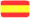SPANISCH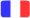FRANZÖSISCH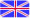ENGLISCH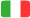ITALIENISCH